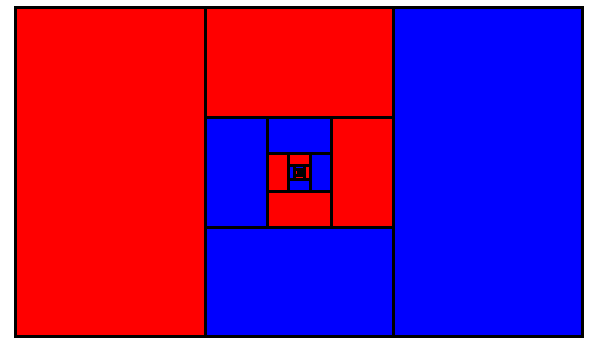# Cutting CakeIn the College Mathematics Journal in 2001, Rick Mabry published this “proof without words” that$\displaystyle \frac{1}{3} + \frac{1}{3^{2}} + \frac{1}{3^{3}} + \cdots = \frac{1}{2}.$

He gives a charming explanation here.

(Rick Mabry, “Mathematics Without Words,” College Mathematics Journal 32:1 [January 2001], 19.)